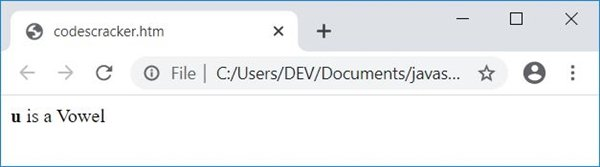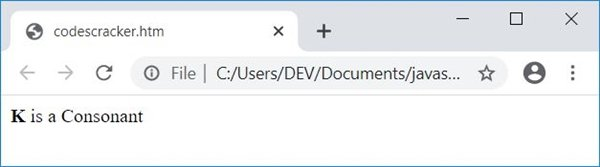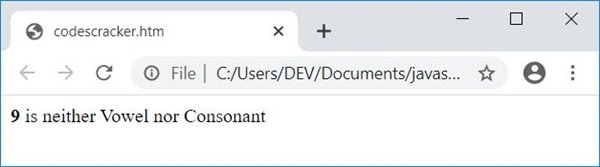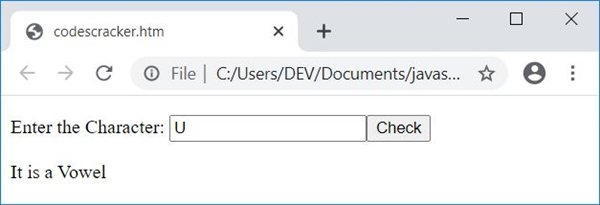# JavaScript Program to Check Vowel or Consonant

In this article, you will learn and get code in JavaScript to check whether a character entered by the user is a vowel or consonant. Here is the list of JavaScript programs available in this article:

• Simple JavaScript Code with HTML to Check Vowel or Consonant
• Modified version of the above program to handle an invalid character
• Allow the user to enter the character and then check for a vowel or consonant.

## Check the vowel or consonant in JavaScript

This is the simplest JavaScript code to check for vowels. That is, this program checks the value stored in the ch variable to see whether it is a vowel or consonant.

```<!doctype html>
<html>
<body>
<script>
var ch='c';
if(ch=='a' || ch=='e' || ch=='i' || ch=='o' || ch=='u')
document.write("<b>"+ch+"</b>" + " is a Vowel");
else if(ch=='A' || ch=='E' || ch=='I' || ch=='O' || ch=='U')
document.write("<b>"+ch+"</b>" + " is a Vowel");
else
document.write("<b>"+ch+"</b>" + " is a Consonant");
</script>
</body>
</html>```

Save this code in a file with .html extension. Open the file in a web browser. Here is the output you will see:Now replace the value of ch with K. That is, initialize K to ch and re-open the file or refresh the web browser. Here is the output:Note: The document.write() method writes into an HTML output.

### Program to handle an invalid character

If the user enters a number or any special character, then the previous JavaScript program produces the output as a consonant, which is the wrong output.

So to handle these types of characters, here is the modified version of the above JavaScript program to check whether a vowel is a consonant or any other character:

```<!doctype html>
<html>
<body>
<script>
var ch='9';
if((ch>='a' && ch<='z') || (ch>='A' && ch<='Z'))
{
if(ch=='a' || ch=='e' || ch=='i' || ch=='o' || ch=='u')
document.write("<b>"+ch+"</b>" + " is a Vowel");
else if(ch=='A' || ch=='E' || ch=='I' || ch=='O' || ch=='U')
document.write("<b>"+ch+"</b>" + " is a Vowel");
else
document.write("<b>"+ch+"</b>" + " is a Consonant");
}
else
document.write("<b>"+ch+"</b>" + " is neither Vowel nor Consonant");
</script>
</body>
</html>```

Here is a sample run of this program with user input 9:### Receive Character Input from the User

This is the complete version of checking a vowel or consonant using the JavaScript program. This program receives character input from the user through an HTML text box. Then it checks and prints whether it is a vowel, consonant, or any other character, as shown in the program given below:

```<!doctype html>
<html>
<script>
var ch;
function checkVowel()
{
ch = document.getElementById("char").value;
if(ch)
{
temp = document.getElementById("resPara");
temp.style.display = "block";
if((ch>='a' && ch<='z') || (ch>='A' && ch<='Z'))
{
if(ch=='a' || ch=='e' || ch=='i' || ch=='o' || ch=='u')
document.getElementById("res").innerHTML = "a Vowel";
else if(ch=='A' || ch=='E' || ch=='I' || ch=='O' || ch=='U')
document.getElementById("res").innerHTML = "a Vowel";
else
document.getElementById("res").innerHTML = "a Consonant";
}
else
document.getElementById("res").innerHTML = "neither Vowel nor Consonant";
}
}
</script>
<body>

<p>Enter the Character: <input id="char"><button onclick="checkVowel()">Check</button></p>
<p id="resPara" style="display:none;">It is <span id="res"></span></p>

</body>
</html>```

Here is its sample output:Now supply any character, sayU, as input and click on the "Check" button. Here is the output produced after performing these things:The following code:

`style="display:none;"`

is a CSS code used to hide an HTML element where it is present.

When the user clicks on the button "Check," the function checkVowel() gets called. The following JavaScript statement:

`ch = document.getElementById("char").value;`

states that the value of an HTML element whose id is char gets initialized to ch. And the following JavaScript code:

`document.getElementById("res").innerHTML = "a Vowel";`

states that the value a Vowel gets written as an HTML element's output whose id is res.

#### Live Output of the Above Program

Here is the live output produced by the previous JavaScript program that was created to check whether the character entered (given) by the user is a vowel, consonant, or any other.

Enter the Character:

JavaScript Online Test

« Previous Program CodesCracker »